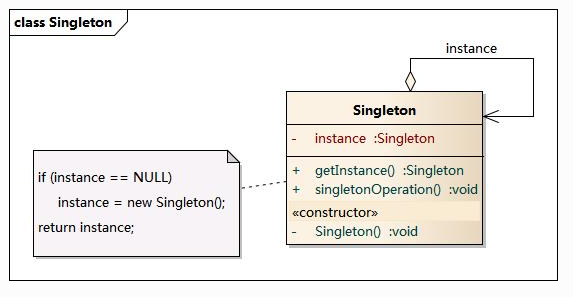# C++软件设计模式之Singleton• windows的Recycle Bin（回收站）也是典型的单例应用。在整个系统运行过程中，回收站一直维护着仅有的一个实例。
• 网站的计数器，一般也是采用单例模式实现，否则难以同步。
• 应用程序的日志应用，一般都何用单例模式实现，这一般是由于共享的日志文件一直处于打开状态，因为只能有一个实例去操作，否则内容不好追加。
• Web应用的配置对象的读取，一般也应用单例模式，这个是由于配置文件是共享的资源。
• 在数据库连接池的设计一般也是采用单例模式，因为数据库连接是一种数据库资源。数据库软件系统中使用数据库连接池，主要是节省打开或者关闭数据库连接所引起的效率损耗，这种效率上的损耗还是非常昂贵的，因为何用单例模式来维护，就可以大大降低这种损耗。
• 多线程的线程池的设计一般也是采用单例模式，这是由于线程池要方便对池中的线程进行控制。
• 操作系统的文件系统，也是大的单例模式实现的具体例子，一个操作系统只能有一个文件系统 上述内容来自于： 单例模式的常见应用场景

• 资源共享的情况下，避免由于资源操作时导致的性能或损耗等。如上述中的日志文件，应用配置
• 控制资源的情况下，方便资源之间的互相通信。如线程池等。

## 如果不使用单例模式是什么样子呢？

#include <cstdlib>  // for rand()
#include <iostream>

class Random {
public:
Random() {
int seed = time(0);
srand(seed);
}
int operator()(int a, int b) const { return (rand() % b) + a; }

private:
Random(const Random&);
Random& operator=(const Random&);
};

int main() {
Random random;
std::cout << random(1, 100) << std::endl;
}


Output:

$./run 44$./run //和第一次间隔时间比较长
72
$./run //后面三个运行程序的速度比较快的话，就会出现得到一样的随机数 44$./run
44
\$./run
44


Function rand () Vs srand()

• rand() function is used in C to generate random numbers. If we generate a sequence of random number with rand() function, it will create the same sequence again and again every time program runs. Say if we are generating 5 random numbers in C with the help of rand() in a loop, then every time we compile and run the program our output must be the same sequence of numbers.
• The srand() function sets the starting point for producing a series of pseudo-random integers. If srand() is not called, the rand() seed is set as if srand(1) were called at program start. Any other value for seed sets the generator to a different starting point. Standard prac tice is to use the result of a call to srand(time(0)) as the seed

## 使用单例模式

• Place constructors and assignment in private section.
• Declare a static instance variable as a data attribute of the class
• Define the static instance variable somewhere in the anonymous namespace.
• Define a static class member function(getInstance) to access the instance variable.

## glut实现模式 (懒汉模式（线程不安全）)

#include <cstdlib>  // for rand()
#include <iostream>

class Random {
public:
static Random* getInstance(); //Define a static class member function
int operator()(int a, int b) const {
return (rand() % b) + a;
}
private:
static Random* instance;  //Declare a static instance variable
Random(const Random&);
Random& operator=(const Random&); // Constructors and assignment in private
Random() {
int seed = time(0);
srand(seed);
}
};
Random* Random::getInstance() {
if ( !instance ) instance = new Random;
return instance;
}
Random* Random::instance = NULL; //Define the static instance variable somewherr

int main() {
Random* random = Random::getInstance();
std::cout << (*random)(1,100) << std::endl;
delete random;
}



volatile T* pInst = nullptr;
T* GetInstance() {
if (nullptr == pInst) {
lock();
if (nullptr == pInst) {
pInst = new T;
}
unlock();
}
return pInst;
}

• if (nulptr == pInst) 中的if 确保仅在 pInst 是空指针的情况下才去获取锁并尝试构造对象；
• if (nullptr == pInst) 的 if 则是为了防止这样一种可能，避免重复操作和内存泄露：在外层 if 检测是，pInst 尚为空，但是，待 lock() 执行完毕后，别的线程已经为 pInst 赋值。

• 为 T 类型的对象分配内存；
• 在这片内存上执行 T 的构造函数；
• 将这片内存的起始地址赋值给 pInst。

#define barrier() __asm__ volatile("mfence")
volatile T* pInst = nullptr;
T* GetInstance() {
if (nullptr == pInst) {
lock();
if (nullptr == pInst) {
T* temp = new T;
barrier();
pInst   = temp;
}
unlock();
}
return pInst;
}


## Meyers单例模式实现

#include <cstdlib>  // for rand()
#include <iostream>

class Random {
public:
static Random& getInstance();
int operator()(int a, int b) const {
return (rand() % b) + a;
}
private:
Random(const Random&);
Random& operator=(const Random&);
Random() {
int seed = time(0);
srand(seed);
}
};
Random& Random::getInstance() {
static Random instance;
return instance;
}

int main() {
Random& random = Random::getInstance();
std::cout << random(1,100) << std::endl;
}


• 懒汉模式申明了一个静态对象，在用户第一次调用时初始化，虽然节约了资源，但第一次加载时需要实例化，反映稍慢一些，而且在多线程不能正常工作。
//First one
Random* Random::getInstance() {
if ( !instance ) instance = new Random;
return instance;
}
Random* Random::instance = NULL; //Define the static instance variable somewherr



• 在类加载时就完成了初始化，所以类加载较慢，但获取对象的速度快。 这种方式基于类加载机制避免了多线程的同步问题，但是也不能确定有其他的方式（或者其他的静态方法）导致类装载，这时候初始化instance显然没有达到懒加载的效果。
//Second One
Random& Random::getInstance() {
static Random instance;
return instance;
}##### Terry Tang
###### Software Development Engineer

My research interests include distributed robotics, mobile computing and programmable matter.